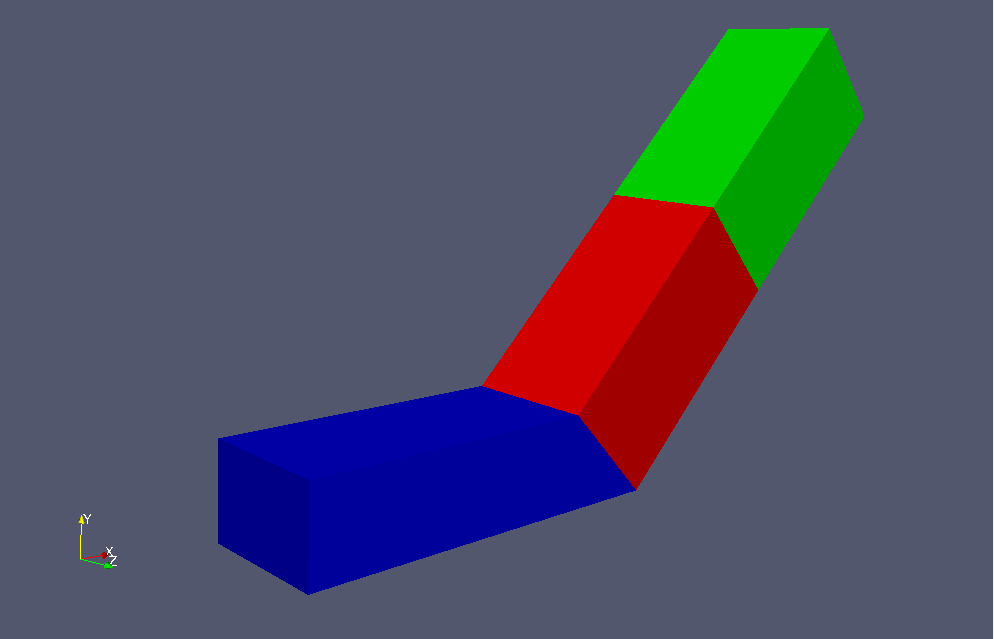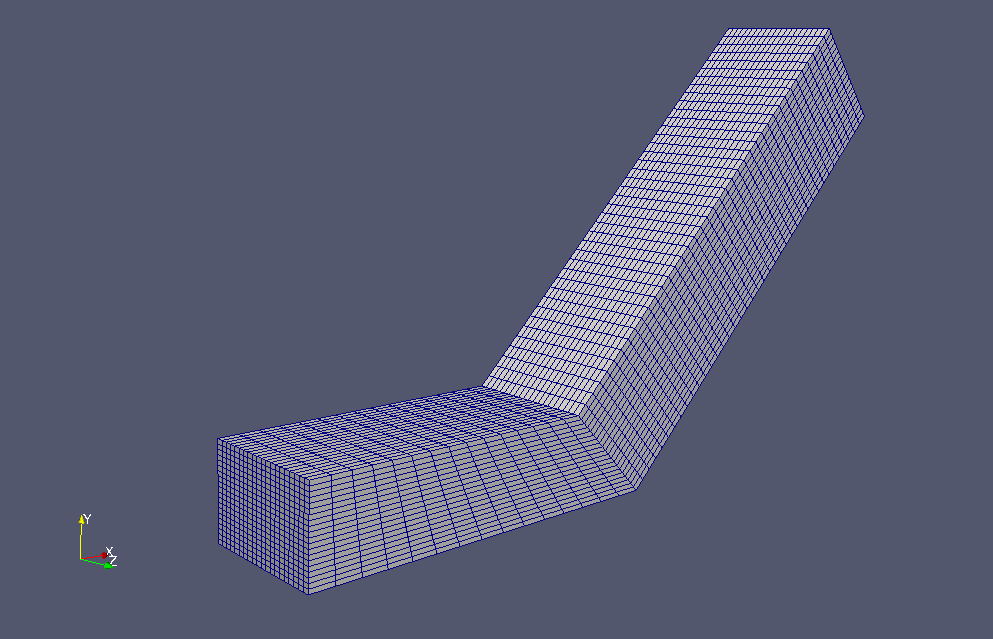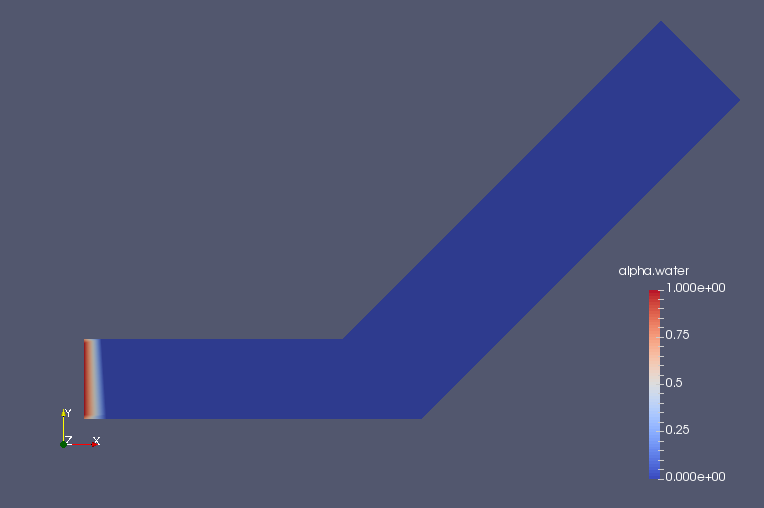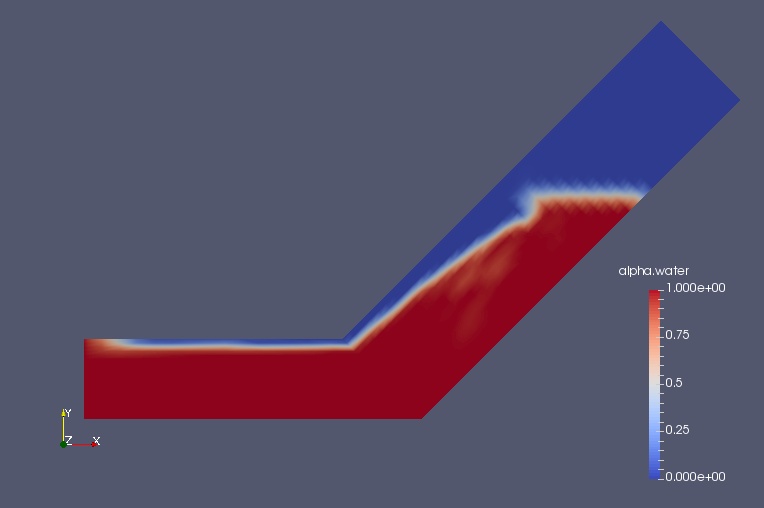﻿ Water inflow into a bending channel with porous media region - XSim

# Water inflow into a bending channel with porous media region

Update: July 1, 2017
OpenFOAM 4.x

$FOAM_TUTORIALS/multiphase/interFoam/ras/angledDuct ## Summary We calculate a flow with a porous material that models a filter in the middle of the flow path. The fluid flows in from the region "inlet" (end face of blue part) at a volumetric flow rate of 0.1 m3/s, passes through the filter (red part), and flows out from the region "outlet" (end face of green part).Model geometry Porous media depends on the Darcy-Forchheimer law, and for a flow velocity ui (i=x, y, z) in direction i, a generation term Si (pressure drop) in the opposite direction of flow is added to the Navier-Stokes equations. Here, μ is the viscosity coefficient and ρ is the density. The parameters that determine the properties of the porous media, Dij, F, the direction of the properties, and the region in which the porous media, are specified in the file "constant/fvOptions" as follows. porosity1 { type explicitPorositySource; active yes; explicitPorositySourceCoeffs { selectionMode cellZone; cellZone porosity; type DarcyForchheimer; DarcyForchheimerCoeffs { d (2e8 -1000 -1000); f (0 0 0); coordinateSystem { type cartesian; origin (0 0 0); coordinateRotation { type axesRotation; e1 (0.70710678 0.70710678 0); e2 (0 0 1); } } } } }  The standard k-ε model is used for the turbulence model. The meshes are as follows, and the number of mesh is 22000.Meshes The calculation result is as follows. Volume ratio of water (alpha.water)Volume ratio of water at initial time (alpha.water)Volume ratio of water at initial time at 4.5 sec (alpha.water) We can see that the water soaks into the porous material and rises up the channel, and after it leaves the porous material, it forms a water surface. When the water surface reaches the bottom of the channel outlet, the water surface stops rising. ## Commands cp -r$FOAM_TUTORIALS/multiphase/interFoam/ras/angledDuct angledDuct
cd angledDuct

m4 system/blockMeshDict.m4 > system/blockMeshDict
blockMesh
interFoam

paraFoam

## Calculation time

5 minutes 43.57 seconds *Single, Inter(R) Core(TM) i7-2600 CPU @ 3.40GHz 3.40GHz